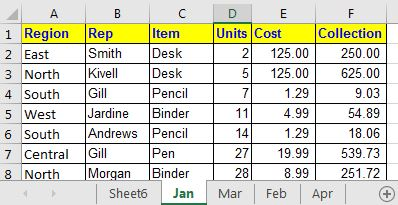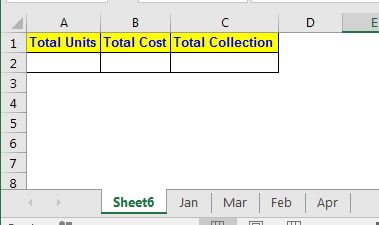3D Reference in Excel

What are 3D references in excel?

Imagine that you have to get the sum of range A2:A10 of 5 multiple sheets in one formula. In that case, you need a formula that not only sums the given range but sums all ranges in given sheets. Here, you need a 3D referencing of excel.

A 3D reference in Excel is the reference that covers identical ranges in multiple sheets. One example is (sheet1:sheet5!A2:A10).

Generic formula to sum a 3D range:

 =SUM(start_sheet:end_sheet! range)

start_sheet: This is the name of the first sheet from which you want to start the sum.

end_sheet:  This is the name of the end sheet. This sheet must not come before the first sheet in the arrangement. The formula will include only the sheets between start_sheet and end_date.

range: It is the range that you want to sum from all the given sheets.

Let's see a 3d reference of excel in action to learn how to use it.

Example: Sum the same range from different sheets using 3-d references in excelIn this example, I have five different sheets that contain similar data. Each sheet contains a month's data. In Sheet6, I want the sum of units, cost, and collection from all the sheets. Let's do it for Units first. The units are in range D2:D14 in all the sheets. Using the 3D referencing of excel write this formula:

 =SUM(Jan:Apr!D2:D14)This returns the value 1384. You can check if it is correct by manually summing all the sheets.

Write similar formulas for each variable.

Note: The 3D referencing can be used where the reference is fixed. It does not work with SUMIF function. We have another workaround for 3D SUMIF.

How does it work?

This formula works in one shot. There are no steps. I guess that the excel loop through each sheet and looks at the same reference to do the calculation.

So yeah guys, this 3D referencing in excel. You can use it where you have fixed cells and formats that don't change their location on the sheet. I hope I was explanatory enough. If you have any query or input to this article or any other Excel/VBA related, put it in the comments section below. We will appreciate it.

Related Articles:

Relative and Absolute Reference in Excel | Referencing in excel is an important topic for every beginner. Even experienced excel users do mistakes in referencing.

Dynamic Worksheet Reference | Give reference sheets dynamically using the INDIRECT function of excel. This is simple...

Expanding References in Excel | The expanding reference expands when copied down or rightwards. We use the \$ sign before the column and row number to do so. Here is one example...

All About Absolute Reference | The default reference type in excel is relative but if you want the reference of cells and ranges to be absolute use the \$ sign. Here's all the aspects of absolute referencing in Excel.

Popular Articles:

50 Excel Shortcuts to Increase Your Productivity | Get faster at your task. These 50 shortcuts will make you work even faster on Excel.

The VLOOKUP Function in Excel | This is one of the most used and popular functions of excel that is used to lookup value from different ranges and sheets.

COUNTIF in Excel 2016 | Count values with conditions using this amazing function. You don't need filter your data to count specific value. Countif function is essential to prepare your dashboard.

How to Use SUMIF Function in Excel | This is another dashboard essential function. This helps you sum up values on specific conditions.

Terms and Conditions of use

The applications/code on this site are distributed as is and without warranties or liability. In no event shall the owner of the copyrights, or the authors of the applications/code be liable for any loss of profit, any problems or any damage resulting from the use or evaluation of the applications/code.